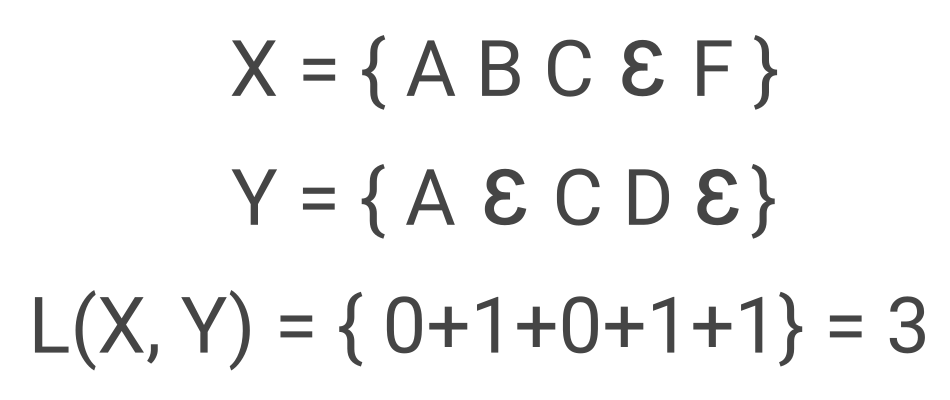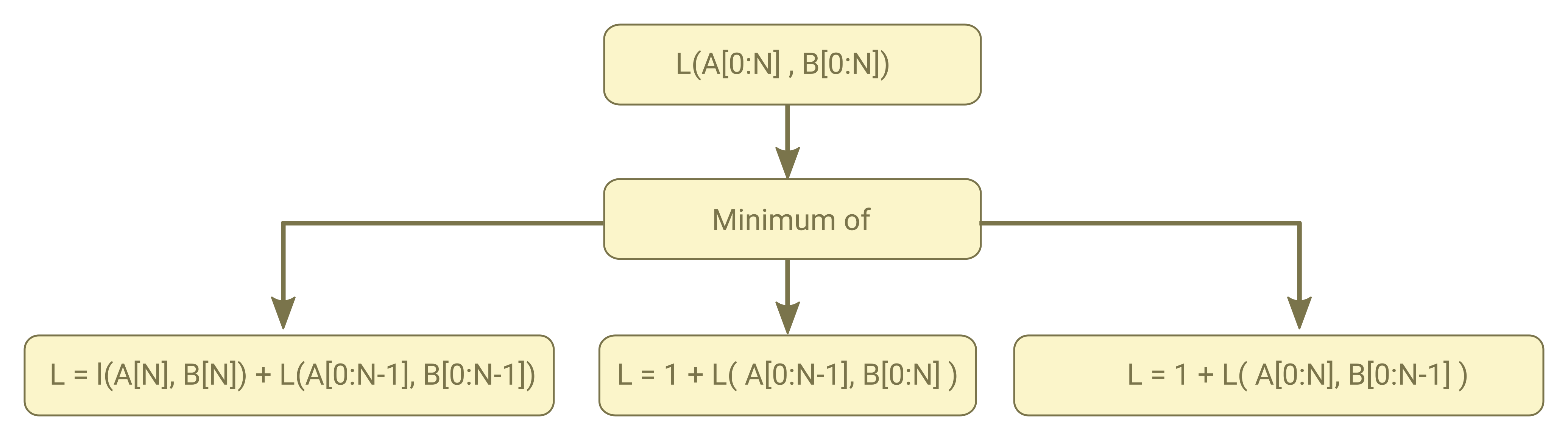To calculate word level confidence score Kaldi uses a method called MBR Decoding. MBR Decoding is a decoding process that minimize word level error rate (instead of minimizing the whole utterance cost) to calculate the result. This may not give the accurate result but can be use to calculate the confidence score up to some level. Just don’t expect too much as the performance is not well-accurate.

Here are some key concepts:

1. Levenshtein Distance: Levenshtein Distance or Edit Distance compute difference between two sentences. It computes how many words are different between the two. Lets say X and Y are two word sequence shown below. The Levenshtein distance would be 3 where Ɛ represent empty wordTo calculate the Levenshtein distance you can use following recursive algorithm where A and B are word sequence with length of N+1As in all recursive algorithm to decrease amount of duplicate computation Kaldi used the memoization technique and store the above three circumstances in a1, a2 and a3 respectively

2. Forward-Backward Algorithm: Lets say you want to calculate the probability of seeing a waveform(or MFCC features) given a path in a lattice (or on HHM FST). Then the Forward-Backward Algorithm is nothing more than a optimized way to compute this probability.3. Gamma Calculation: TBA

4. MBR Decoding: TBA

Recent Posts